基于灰色理论下的城市空气污染状况研究及成因分析Urban Air Pollution Research and Its Cause Analysis Based on Gray Theory

• 全文下载: PDF(681KB)    PP.154-163   DOI: 10.12677/SA.2018.72019
• 下载量: 413  浏览量: 1,147   科研立项经费支持

In this paper, a gray prediction GM (1,1) model and a BP neural network model are respectively used to predict the air quality status of all first-tier cities and new-tier cities in China. From the better predictions, six cities that cannot meet the air quality standards set by the 13th Five-Year Plan of China by 2030. They are Beijing, Wuhan, Tianjin, Chongqing, Xi’an and Changsha. Then, the air quality status and air pollutants of the above six cities were analyzed by gray relational analysis. Therefore, we identify the main air pollutants in each city. Finally, the main pollutants are integrated using principal component analysis and multiple linear regression to find out the main causes of the major pollutants in each city and provide an important reference for the en-vironmental governance in each city.

1. 引言

“十一五”期间，我国环境保护取得了令人可喜的成绩，特别是空气污染物这项指标超额完成规划目标。但是我国环境形势任然很严峻，根据耶鲁大学公布的全球环境绩效指数(EPI)报告显示，中国GDP总量从2006年的第四名上升到目前第二名并稳居第二名，然而环境绩效指数却从2006年的第94名(参评133个国家)下降到2016年的倒数第2名，其中空气质量是拖后中国排名的最主要的原因   。中国用40年的时间基本上完成了发达国家100多年的城市化进程，却也只用30多年的时间“集聚”和“爆发”了发达国家100多年的环境问题。如果中国解决不好当前我国的环境问题，不但会严重影响社会经济的可持续发展，还可能影响到政府执政的公信力。

2. 各大城市大气污染物浓度及其趋势预测

2.1. 各大城市大气污染物浓度值Table 1. The concentration of air pollutants in Beijing in recent years (excerpt)

2.2. 各大城市按照其污染程度分类

AQI (Air Quality Index)是空气质量指数定量描述空气质量状况的无量纲指数。其数值越大、级别和类别越高，说明空气污染状况越严重，对人体的健康危害也就越大。

AQI的计算公式如下：

$AQI=\mathrm{max}\left\{IAQ{I}_{1},IAQ{I}_{2},\cdots ,IAQ{I}_{n}\right\}$

$IAQ{I}_{p}=\left(IAQ{I}_{Hi}-IAQ{I}_{Lo}\right)×\left({C}_{P}-B{P}_{Lo}\right)/\left(B{P}_{Hi}-B{P}_{Lo}\right)+IAQ{I}_{Lo}$

IAQIP——污染物项目P的空气质量分指数；

CP——污染物项目P的质量浓度值；

BPHi——相应地区的空气质量分指数及对应的污染物项目浓度指数表中与CP相近的污染物浓度限值的高位值；

BPLo——相应地区的空气质量分指数及对应的污染物项目浓度指数表中与CP相近的污染物浓度限值的低位值；

IAQIHi——相应地区的空气质量分指数及对应的污染物项目浓度指数表中与BPHi对应的空气质量分指数；

IAQILo——相应地区的空气质量分指数及对应的污染物项目浓度指数表中与BPLo对应的空气质量分指数。

2.3. 各大城市空气质量指数以及各大气污染物浓度的预测

2.3.1. 灰色预测Table 2. AQI’s relation to the classification of air quality

2.3.2. BP神经网络预测

2.3.3. 灰色预测与BP神经网络预测误差比较分析

2.3.4. 至2030年未能达标的城市

2.4. 未达标城市的污染物与空气质量指数的灰色关联度分析

2.4.1. 灰色关联度模型

STEP1：选取参考序列，这里以AQI的数据为参考序列。

${x}_{0}=\left\{{x}_{0}\left(k\right)|k=1,2,\cdots ,n\right\}=\left({x}_{0}\left(1\right),{x}_{0}\left(2\right),\cdots ,{x}_{0}\left(n\right)\right)$ (k表示时刻， $n=7$ ) (1)

${x}_{i}=\left\{{x}_{i}\left(k\right)|k=1,2,\cdots ,n\right\}=\left({x}_{i}\left(1\right),{x}_{i}\left(2\right),\cdots ,{x}_{i}\left(n\right)\right),\text{\hspace{0.17em}}i=1,2,\cdots ,m$ (m为比较数列的个数) (2)

STEP2：比较各污染物的数据对AQI在各时刻的关联系数

${\xi }_{i}\left(k\right)=\frac{\underset{s}{\mathrm{min}}\underset{t}{\mathrm{min}}|{x}_{0}\left(t\right)-{x}_{s}\left(t\right)|+\rho \underset{s}{\mathrm{max}}\underset{t}{\mathrm{max}}|{x}_{0}\left(t\right)-{x}_{s}\left(t\right)|}{|{x}_{0}\left(k\right)-{x}_{i}\left(k\right)|+\rho \underset{s}{\mathrm{max}}\underset{t}{\mathrm{max}}|{x}_{0}\left(t\right)-{x}_{s}\left(t\right)|}$ (3)

STEP3：计算各污染物的数据对AQI的关联度。

${r}_{i}=\frac{1}{n}\underset{k=1}{\overset{n}{\sum }}{\xi }_{i}\left(k\right)$ (4)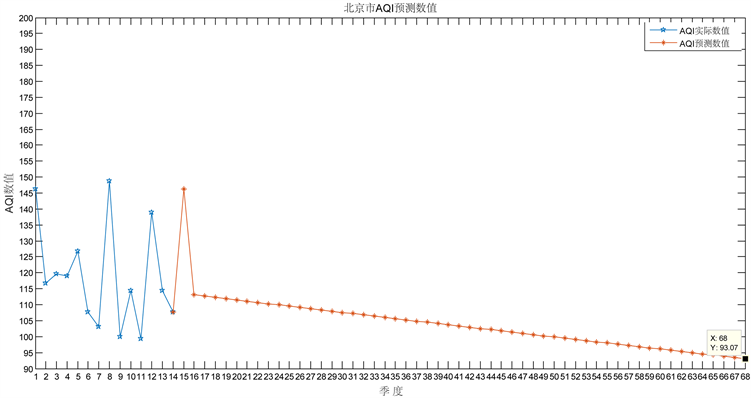Figure 1. AQI grey prediction results of Beijing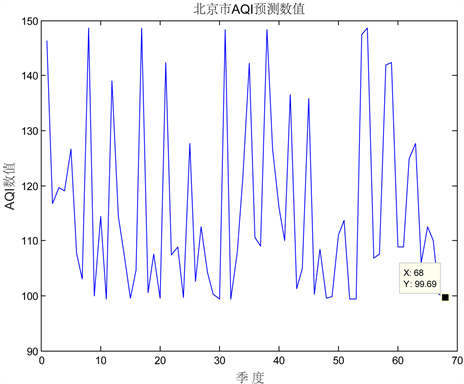Figure 2. BP neural network prediction results of AQI in Beijing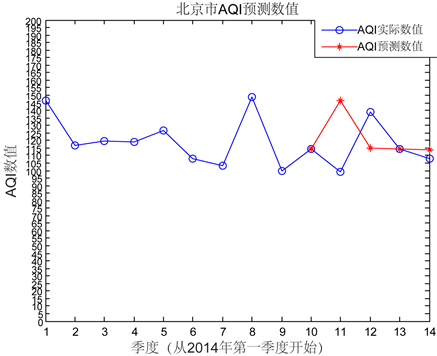Figure 3. The error test of grey prediction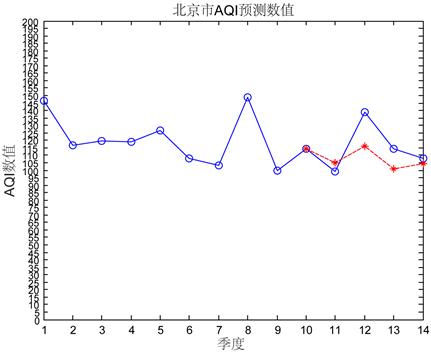Figure 4. Error test of BP neural network

2.4.2. 未达标6座城市各污染物浓度与AQI的关联度

3. 未达标城市的主要污染物的成因分析

3.1. 污染物成因的指标的确定Table 3. Correlation between the concentration of pollutants and AQI in 6 cities

3.2. 各城市主要污染物的主要成因分析

3.2.1. 最小二乘回归

$\mathrm{min}{\left(\stackrel{^}{Y}-Y\right)}^{2}$

$y=1175.97+0.018{x}_{1}+0.043{x}_{2}+0.13{x}_{3}-3488.32{x}_{4}+0.0004{x}_{5}+245.19{x}_{6}$

$Rms{e}_{1}=14.0524$

3.2.2. 主成分分析

$y=151.78-0.001{x}_{1}-0.002{x}_{2}-0.07{x}_{3}-260.63{x}_{4}+0.0006{x}_{5}+151.05{x}_{6}$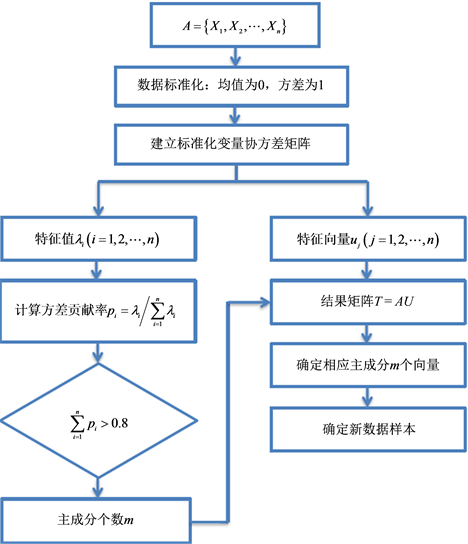Figure 5. Flow diagram of principal component analysis

$Rms{e}_{2}=13.3811$

3.2.3. 综合分析

$y=1531.01+0.06{x}_{1}-0.06{x}_{2}-0.0001{x}_{3}-417.46{x}_{4}-0.0002{x}_{5}-0.0001{x}_{6}$

$y=145.58-0.000435{x}_{1}-0.0015{x}_{2}-13.83{x}_{4}-0.0003{x}_{5}+0.2032{x}_{6}$

$y=699.45+0.25{x}_{1}+0.067{x}_{2}+1.33{x}_{3}-10.19{x}_{4}-0.03{x}_{5}+1.29{x}_{6}$

$y=312.29+0.004{x}_{1}+0.002{x}_{2}-6.64{x}_{4}-0.001{x}_{5}+2.14{x}_{6}-11.51{x}_{7}$

$y=6.36-0.003{x}_{1}-140.72{x}_{4}+0.58{x}_{6}-19.31{x}_{7}$

4. 结语

1) 华中师范大学2017年度大学生创新创业训练计划B类项目；

2) 上海市青锐环境数据有限公司科研立项资助。

1、附件1：各一线城市和新一线城市各季度空气污染物数据统计表： https://pan.baidu.com/s/1oDAsS3t2YM0xT2nDQAQjTA

2、附件2：各城市空气质量状况预测结果汇总(图像需用MATLAB打开)：

https://pan.baidu.com/s/1kAs2o4NEgtG7D7hOLTcXVQ

3、附件3：6座未达标城市的城市环境统计年鉴网址：

  Dennis, R.L., Byun, D.W., Novak, J.H., Galluppi, K.J., Coats, C.J. and Vouk, M.A. (1996) The Next Generation of Integrated Air Quality Modeling: EPA’s Models-3. Atmospheric Environment, 30, 1925-1938. https://doi.org/10.1016/1352-2310(95)00174-3  刘道文, 忽海娜. 非线性时间序列混沌特性分析及短期预测[J]. 计算机仿真, 2012, 29(10): 370-373.  邓聚龙. 灰色系统基本方法[M]. 上海: 华中理工大学出版社, 1988: 58-76.  孙中党, 赵勇, 田超, 李小浩. 郑州大气氮氧化物污染与灰色分析[J]. 重庆环境科学, 2003(1): 52-55, 62.  王彩琴, 朱桦. 大气污染灰色预测的应用研究[J]. 西安建筑科技大学学报(自然科学版), 1998(1): 58-62.  樊庆锌, 刘沙沙, 于淼. 灰色模型理论在哈尔滨市大气污染物NO2预测中的应用[J]. 环境保护科学, 2008(5): 1-3, 6.  刘阳荷. 中国大气污染物排放量变动影响因素分析与精准减排[D]: [博士学位论文]. 济南: 山东大学, 2017.  王海林. 北京市PM2.5/PM10的源解析[C]//中国化学会. 中国化学会第26届学术年会环境化学分会场论文集: 2008年卷. 中国化学会, 2008: 1.  石灵芝, 邓启红, 路婵, 刘蔚巍. 基于BP人工神经网络的大气颗粒物PM_(10)质量浓度预测[J]. 中南大学学报(自然科学版), 2012, 43(5): 1969-1974.  欧阳钧, 王爱枝. 基于Matlab的BP神经网络在大气污染物浓度预测中的应用[J]. 环境科学与管理, 2009, 34(11): 176-180.  Hedley, M.A. (1997) Evaluation of an Air Quality Simulation of the Lower Fraser Valle Meteorology Photochemistry. Atmospheric Environment, 31, 1605-1630.  Zeng, B. and Li, C. (2016) Forecasting the Natural Gas Demand in China Using a Self-Adapting Intelligent Grey Model. Energy, 112. https://doi.org/10.1016/j.energy.2016.06.090